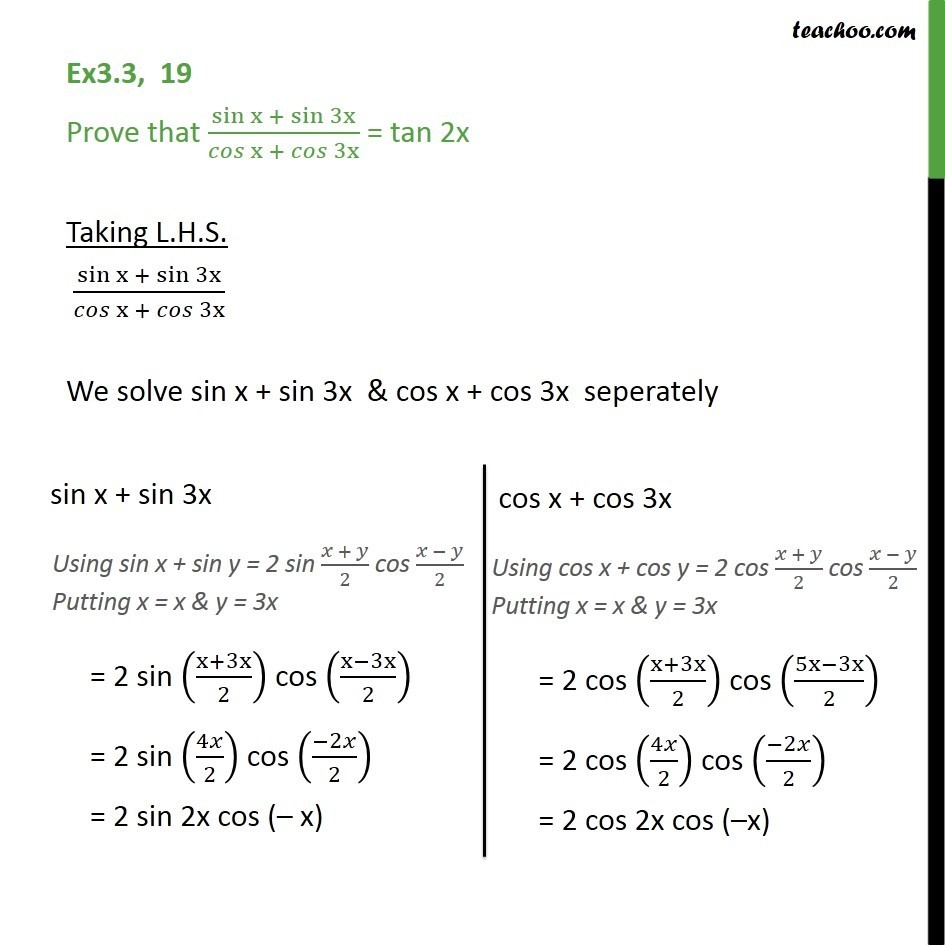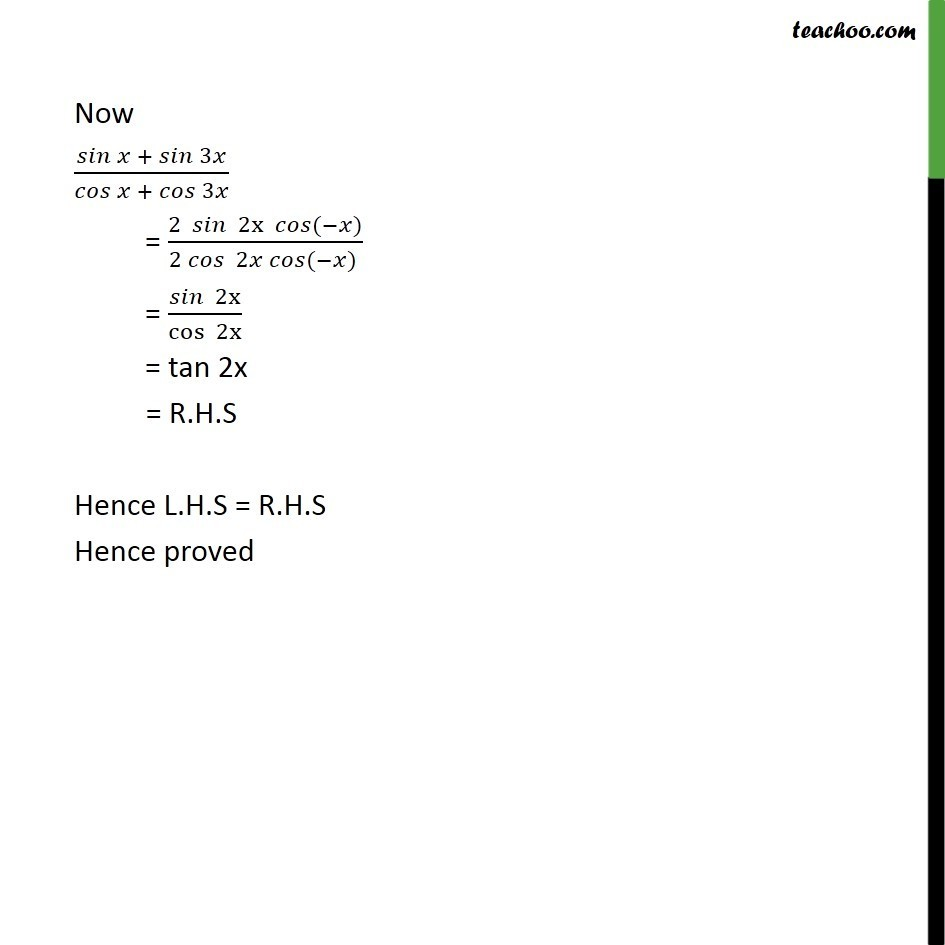Subscribe to our Youtube Channel - https://you.tube/teachoo

1. Chapter 3 Class 11 Trigonometric Functions
2. Concept wise
3. Cos x + cos y formula

Transcript

Ex3.3, 19 Prove that sin x + sin 3x /( x + 3x ) = tan 2x Taking L.H.S. sin x + sin 3x /( x + 3x ) We solve sin x + sin 3x & cos x + cos 3x seperately Now + 3 / + 3 = (2 2x ( ) )/(2 2 ( ) ) = 2x /cos 2x = tan 2x = R.H.S Hence L.H.S = R.H.S Hence proved

Cos x + cos y formula